### Journal of Genetic Engineering

Current Issue Volume No: 1 Issue No: 1

ISSN: 2694-1198

Mini Research Article Open Access
• Available online freely Peer Reviewed
• ### Inbreeding in a Family Tree and in a Population

A.N. Volobuev 1

1Samara State Medical University, Department of Medical Physics, Samara, Russia

## Abstract

On the basis of Hardy – Weinberg’s law the problem of inbreeding in a family tree and a population was investigated. With use of an inbreeding factor are received the discrete equation for a family tree and differential equation for a population. The numerical solution of the differential equation for a population was found and analyzed at various values of the inbreeding factor. Migration of inbred population is investigated in view of natural selection. It was shown that velocity of migration falls with increase of the inbreeding factor. Interrelation of the recessive allele frequency at woman for a migrating population with inbreeding factor and standard parameter of selection was found.

##### Author Contributions
Received 08 Feb 2020; Accepted 28 Feb 2020; Published 04 Mar 2020;

Academic Editor: Francis Mwesigye, Makerere university, Kampala, Uganda.

Checked for plagiarism: Yes

Review by: Single-blindThis is an open-access article distributed under the terms of the Creative Commons Attribution License, which permits unrestricted use, distribution, and reproduction in any medium, provided the original author and source are credited.

Competing interests

The authors have declared that no competing interests exist.

Citation:

A.N. Volobuev (2020) Inbreeding in a Family Tree and in a Population. Journal of Genetic Engineering - 1(1):21-34.

## Introduction

Problem of inbreeding is one of the major problems of genetics. Since times of the human occurrence the population size was very small. People lived separate tribes. Therefore, consanguineous mating or inbreeding has been submitted enough. In process of the population size increase the intensity of such mating was reduced. But till now the consanguineous mating are present at small communities.

Inbreeding are widely submitted at animals. Very much frequently the man uses consanguineous mating of animals at artificial selection to receive useful properties of animals: speed of run, force, fleshiness, a plenty of milk, a wool, etc.

Let's consider principles of inbreeding in a family trees and a population.

## Inbreeding in a Family Tree

There are family trees and populations where the principle panmictic mating is essentially broken. Usually it is geographically or socially relatively isolated small communities. In these communities the inbreeding i.e. consanguineous mating of various orders (a parent - a child, sibs, cousins, uncle - niece, etc.), can be wide-spread.

The problem of inbreeding influence on the population is the important problem of genetics 1. Now this problem yet has not received the final solution.

In most cases the inbreeding in a family tree take into account on three generations. It is the most distant relatives who are taken into account at consanguineous mating there is second cousins.

The inbreeding is taken into account with the help of inbreeding factor.

The inbreeding factor is the probability two alleles are identical by origin.

Let's consider the method of factor inbreeding factor calculation in a family tree offered by Wright 1, 2.

For the inbreeding factor finding it is necessary:

to find for the marrying descendants all ways in a family tree which through the common ancestor connect these descendants.

to count up number of steps (alternations of generations) on each way.

Then the inbreeding factor is equal: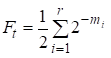……(1)

where r there is number of ways, m1, m2, ... mi- number of steps on each way.

Once again we shall note that the number of addends in the sum (1) is equal to number of ways which through the common ancestors connect marrying.

Let's consider concrete cases of family trees, Figure 1. In this figure the square means a male individual the circle – female individual. Dotted lines and identical digits specify possible ways in a family tree which through the common ancestor (the man or the woman) connect of the descendants consanguineous marrying. On some sites of ways, Figure 1с, there are two digits 1 and 2. It specifies that such sites are the common for the various ways.

The marriage a father - daughter is incest, Figure 1а. The descendant (woman) is connected with the ancestor (man) there 1 way with one step, i.e. m = 1. Hence, there is inbreeding factor.

Ft = 1/2.2-1 = 1/4

There is marriage a brother - sister (sibs), Figure 1b. Digits specify the number of the way. The numbers of identical digits on the way from the marrying man to the woman is equal the number of steps.

Let each descendant who has married connects with partner two ways with two steps on the each way, i.e. m = 2. Hence, there is inbreeding factor.

Ft = 1/2.(2-2 + 2-2) = 1/4

The marriage between cousins is shown on Figure 1с.

Figure 1. Some kinds of inbreeding in a family tree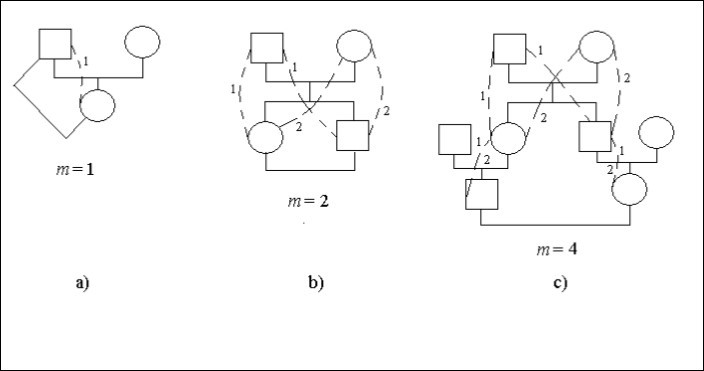Each descendant who has married connects with partner the 2 ways with 4 steps on the each way, i.e. m = 4. Hence, there is inbreeding factor.

Ft = 1/2.(2-4 + 2-4) = 1/16

The formula (1) is easy for understanding from the following logic.

Obviously there 1/2 genes of the child is the common with each parent, 1/4 - with the grandfather and grandmother, 1/8 - with the great-grandfather and great-grandmother, etc. If from the woman to any ancestor leads the way with η steps the share of the common genes with this ancestor is equal 2-η. If from the man to any ancestor leads the way with μ steps the share of the common genes is equal 2-μ. At the marriage of the man and woman with the common ancestor the share of the common genes with this ancestor will be 2-η. 2-μ = 2-m where m = η + μ there is total number of steps through the common ancestor from the woman up to the man marrying. The size 1/2 2-m shows probability that the gene casually chosen at the woman will be identical by origin to the gene casually chosen at the man. The same size gives the contribution to the inbreeding factor Ft one way through the common ancestor from the woman up to the man marrying.

For example, if the common ancestor will be away from marrying on η = μ = 5 generations the probability them to have the common gene is equal. 1/2 2-(5+5) = 1/2048 This size at the analysis of mating can be neglected. (Table 1)

Table 1. inbreeding factors for the some consanguineous marriages are shown in addition.
 The kind of consanguineous marriage Inbreeding factor Ft Second cousins 1/32 Cousin uncle - niece 1/32 Third cousins 1/64

The inbreeding factor Ft is the probability of two alleles in posterity are identical by origin i.e. occur from same gene of ancestor 1. Or, that too most, the inbreeding factor is a probability of the allele origin in posterity is consanguineous. Hence, the probability of the non-consanguineous origins of identical alleles in homologous chromosomes is equal 1 - Ft.

Let's find total probability of the recessive homozygote aa occurrence (eventаа) at random mating for an autosomal genes 3: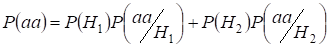.......(2)

This formula follows from the following logic of mating: the origin of identical alleles in homologous autosomes is non-consanguineous (the probability of this hypothesis is P(H1) = 1– Ft )also arises homozygoteаа (conditional probability of this event is P(aa/H1) = q2) or origin of identical alleles is consanguineous (the probability of this hypothesis is P(H2) = Ft ) and arises homozygoteаа (conditional probability of this event is P(aa/H2) = q). The probability q of the homozygote aa occurrence in the latter case is determined by that if allele a is in one autosome then at consanguineous origin of homozygote same allele necessarily should be and in the homologous autosome.

Hence, the total probability of the recessive homozygote occurrence is equal: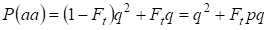……….(3)

where it is taken into account p = 1 - q there is the dominant allele a frequency.

Similarly there find total probability of the dominant homozygote AA occurrence at random mating: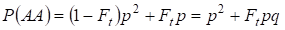……….. (4)

Thus, according to (3) and (4) total frequency of homozygotes (dominant and recessive) in inbred family tree increases on 2Ftpq. Hence, frequency of heterozygotes decreases for the same size and to become equal 2pq - 2pqFt = 2pq(1-Ft) since the sum of the all zygote frequencies is equal 1.

In view of inbreeding factor and also (3) and (4) the Hardy - Weinberg law in the following generation of inbred family tree for an autosomal genome needs to be written down the distributions of zygote genotypes as 4: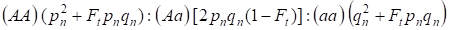…………(5)

Where pn is the dominant alleles A frequency in generation n, qn - the recessive alleles a frequency, so pn + qn = 1.

The ratio (5) refers to also Wright’s ratio 5. Wright’s ratio shows that in an inbred family tree after the consanguineous mating of individuals are observed the following effects: there is increase in the autosomal homozygotes fraction in a family tree on Ft pnqnand reduction of the autosomal heterozygotes fraction in (1-F1) ones in comparison with a panmictic population.

But already through one generation the Hardy – Weinberg balance is restored that specifies the small importance of the separate consanguineous mating for a family tree: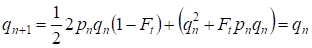.........(6)

Obviously, for a population as whole the separate act of the consanguineous mating will have still smaller importance than for a family tree.

The same effect of variation of zygote genotypes frequency is observed and for sex-linked genes.

Distribution of the genotypes linked tothe Х-chromosome in panmictic family tree is described by Hardy – Weinberg law as 1: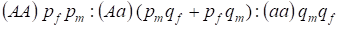………..(7)

The frequency of a dominant alleles A we shall designate at the men pm and at the women pf. For recessive allelesa it is accordingly qm and qf .

At mating there is a ratio of genotypes at the women according to product (pf + qf) (pm +qm).

Let's find the total probability of the recessive homozygote aa occurrence at random mating for the daughter’s genes linked to theХ-chromosome under the formula (2). The logic of events is similar to the case for autosomes except that the conditional probability of a genotypeаа occurrence from the non-consanguineous alleles according to (7) is equal P(aa/H1) = qmnqfn. For the consanguineous alleles the conditional probability of a genotypeаа occurrence is equal P(aa/H2) = qf(n+1). At daughters, i.e. in generation n+1 the probability of a homozygote aa occurrence is determined by that if allele a is in one Х-chromosome then at consanguineous origin of homozygote same allele necessarily should be and in the homologous Х-chromosome.

Hence, the total probability of the recessive homozygote occurrence is equal: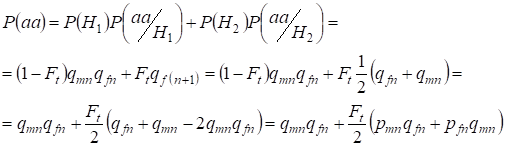……(8)

where pmn is the dominant alleles A frequency in generation n at men and pfn - at women. For the recessive alleles a accordingly qmn and qfn . Besides it is used qmn =1 - qmn and pfn = 1 - qfn.

Similarly finding the total probability of the dominant homozygote occurrence with use: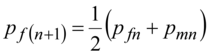,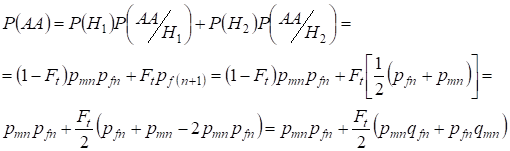……….(9)

and a heterozygote P(Aa) = 1– P(aa) - P(AA) we shall find distribution of the genotypes in Х-chromosomes of the inbred family tree at mating:……..(10)

It is simultaneously demonstrated that as well as in autosomes the inbred additives in dominant and recessive homozygote frequencies in the sex-linked chromosomes are identical.

Other way of the ratio (10) finding will consist in the following.

Let's find first the total probability of a heterozygote Aa occurrence, i.e. P(Aa) at the opportunity of the random consanguineous mating. This probability follows from the following logic of mating: the origin of alleles is non-consanguineous (the probability of this hypothesis is P(H1) = 1 - Ft ) also arises heterozygote Aа (conditional probability of this event is P(Aa/H1) = pmnqfn + pfnqmn, see the formula (7)) or origin of alleles is consanguineous (the probability of this hypothesis is P(H2) = Ft) and arises heterozygote Aа (conditional probability of this event is equal to zero P(Aa/H2) = 0 ). Last statement is defined by that at consanguineous origin similar alleles in homologous Х-chromosomes cannot the heterozygoteАа arise consisting different alleles.

Hence, using the formula of total probability we find total probability of the heterozygote Aa occurrence: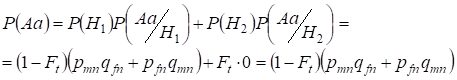……..(11)

Reduction of a heterozygotes Aa frequency occurs as has been proved earlier due to identical increase in dominant AA and recessive aa homozygotes frequency. For example, the increase in recessive aa homozygotes frequency is equal: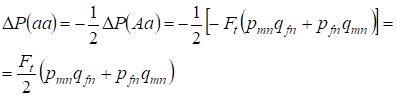……..(12)

where reduction of the heterozygotes Aa frequency relative of panmictic family tree according to (7) and (11) is equal. ΔP(Aa) = - Ft (pmnqfn + pfnqmn) Using (11) and (12) to update (7) for cases of inbreeding we find the distribution of genotypes (10) in Х-chromosomes in the daughters inbred family tree.

In following generation the frequency of the recessive alleles a at women is equal: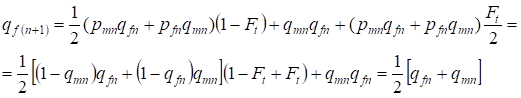……..(13)

That fully complies with same frequency at absence of inbreeding, i.e. there is restoration of the Hardy – Weinberg balance or is more exact approach to the balance.

The ratio (7) and (13) show as a whole the allele frequencies in a family tree for the account of inbreeding do not vary. There is only an alleles redistribution. A part of alleles leave the heterozygotes and pass to the homozygotes.

## Genetic Load in a Family Tree

In the inbred populations so-called the genetic load frequently connected to harmful mutations only recessive alleles passed to offspring is accumulates.

It is connected to increase in the recessive homozygotes aa frequency in the inbred populations or family tree, see (3) or (8), in comparison with panmictic. The increase of inbreeding can increase frequency of recessive homozygotes for mutant alleles.

The concept of the genetic load in the population was used for the first time the known geneticist Nobel laureate H. J. Muller 6. However, actually the theory of the genetic load has been developed only for a family tree.

The consanguineous mating in a population increase homozygotes frequency of harmful mutant alleles. Therefore, it is supposed that inbreeding is one of principal causes of occurrence and accumulation of the genetic load.

The genetic load can be expressed in lethal equivalents. The lethal equivalent is, for example, one lethal mutation resulting in death of an individual in all cases or two lethal mutations each of which resulting in death of the individuals in 50% cases, etc.

At research of the genetic load in a family tree the action of selection we shall not take into account to not complicate the analysis. It is allowable since selection submits to other laws rather than occurrence of mutations.

According to the genetic load theory the formula (10) needs to be transformed 1: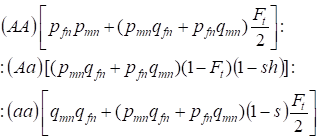, ……...(14)

where s there is probability of the recessive homozygote destruction as consequence of the inbreeding, sh - probability of the heterozygote destruction as consequence of the inbreeding, h - so-called dominant degree of the mutation which have occurred owing to inbreeding. If h = 1 there are heterozygotes and recessive homozygotes perish with the identical frequency, if h = 0 the heterozygotes are resistant to inbreeding. Dominant homozygotes are assumed absolutely resistant to mutations. It is mutational model of process.

Let's notice that the ratio (14) concerns to a family tree as separate usually small making population.

Using (14) we shall find the recessive allele a frequency at women in the following generation: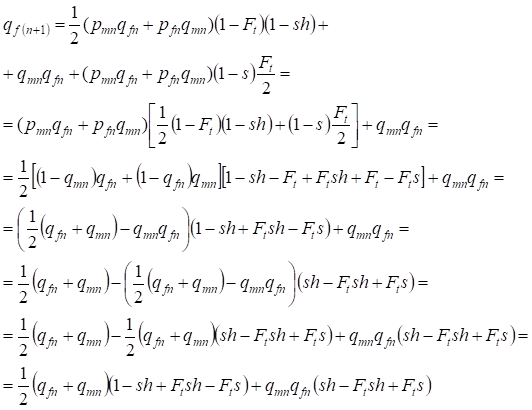…….(15)

In the following generation there is no restoration of the Hardy - Weinberg balance, i.e. accumulation of the genetic load in a family tree, and hence and in a population is observed.

Let's enter the designation F = sh- Ftsh+ Fts - factor of inbreeding for the population. We shall note that if the heterozygotes are resistant to mutations owing to inbreeding h = 0 , and recessive homozygotes are perish with probability s = 1 the inbreeding factors of the populations and family tree are equal F = Ft. Thus the formula (15) can be written down as: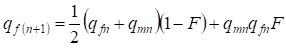……(16)

Lack of the formula (16) is absence normalization on the general fraction of genes less than 1 the rest after lethal mutations. However the standard way of normalization results in too complex formulas which do not allow proceed to the analysis of the population.

For updating the formula (16) it is used the following logic. In the condition of balance of the family tree with inbreeding the formula (13) for calculation of the recessive allele a fraction is correct. The formula (13) is absolutely correct since is normalized on the general portion of genes equal 1.

Taking into account (13), and also F<<1 and small size of the second addend in the right part (16) it is possible to assume that for normalization (16) it is necessary to divide the right part into value 1 - F .

Therefore, the corrected formula (16) looks like: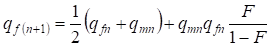……(17)

Accuracy of the formula (17) can be estimated as follows. Using the formula similar (13) for frequency of dominant allele A in generations of women and summing it up with the formula (13) for frequency of recessive allele a we find pf(n+1) + qf(n+1) = 1/2(pfn + qfn + pmn + qmn)=1that it is possible to assume a condition of normalization. If instead of the formula (13) to lead similar transformations with the formula (16) the condition of normalization is not carried out even in the main (first) order on allele frequencies. For the formula (17) condition of normalization in the main order is carried out. The mistake of normalization is O = (pmnpfn + qmnqfn) F/(1 - F) i.e. concerns to higher (second) order of smallness on allele frequencies.

The formula (17) allows find the recessive allele a fraction at women in the following generation of the family tree as the sum of equilibrium addend and gradually increasing owing to inbreeding the genetic load.

## Inbreeding in a Population

Let’s carry out the analysis of the inbreeding action on a population.

At research of inbreeding in populations the action of selection we shall not take into account (it will be taken into account later) to not complicate the analysis. It is allowable since selection submits to other laws rather than occurrence of mutations owing to inbreeding.

Using a standard technique of transition from a family tree to a population 7 in the basis of the analysis we shall put the formula (17) which allows to calculate fraction of the recessive allele a inthe Х-chromosome of women in the following generation as the sum of the equilibrium term and gradually increasing genetic load owing to inbreeding.

Taking into account that the man inheritsthe Х-chromosome from mother i.e. qmn = qf(n-1) it is possible to transform (17) to kind: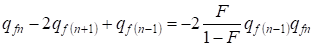……(18)

where F there is inbreeding factor for a population.

The differential equation for change of frequency qfn of recessive allele a in the inbred population we shall write down in general form: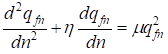, …….(19)

where the independent variable n there is in this case time of the population life normalized on average on family trees of the population time of one alternation generation (approximately 25 years) i.e. actually continuous dimensionless time, η and μ - constants.

Let's pass in (19) to finite-differential form: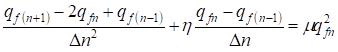……..(20)

Let's collect the like terms: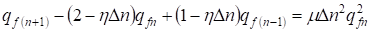……..(21)

Let's multiply (21) on -2 besides we shall replace

that is allowable for continuous in time of the generation alternation scale i.e. for a population: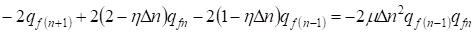………(22)

Let's identify (22) and (18). In result we have system of three algebraic equations: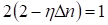……(23)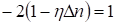……...(24)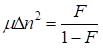……….(25)

The solution of the given system looks like: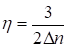, ……...(26)

that similarly the equilibrium panmictic population, 7.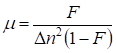…….(27)

Hence, the differential equation (19) can be copied as: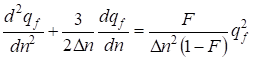…….(28)

The number of generation for the population does not play a role - the continuous time scale therefore, an index n is omitted.

The found nonlinear differential equation (28) is the Hardy-Weinberg law for a population with the account of inbreeding.

At F = 0 (inbreeding is absent) the equation (28) it will be transformed to the kind that corresponds to the equilibrium panmictic population:

We use in (28) new independent variable . t = n/Δn F/(1-F) If to accept 7 Δn = 3/2ln 2

we shall find

For the new variablet the equation (28) depends only on one constant parameter Ԑ: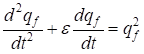…….(29)

where it is designated Ԑ = 3/2 √ (1-F) / F . At variation of the inbreeding factor 0 ≤ F ≤ 1 the size is ∞ ≥ Ԑ ≥ 0.

The nonlinear differential equation (29) can be solved only numerically.

Initial conditions first of all are necessary for the solution of the differential equation (29): initial frequency qf0 of the recessive allele a and initial speed of increase in this frequency (dqf/ dt)0 or (dqf/dn)0.

Initial frequency qf0 depends on time of the beginning of the population analysis. Therefore, without reduction of the analysis generality at t = 0 or n = 0 we shall accept qf0 = 0.6

Various variants of numerical calculations under the formula (29) show that the result of calculation at small initial speed of the frequency increase qf practically does not depend on this speed. Therefore, was accepted. (dqf/ dt)0 = (dqf/dn)0 = 0 The step of the variable t equal Δt = 0.001 and numbers of steps 20000 at calculation were used.

On Figure 2 the calculation of the population dynamics of recessive allele a on the basis of the equation (29) at various inbreeding factors F is shown. On the abscissa axis the size n ≈ t/0.462√(1-F)/F is plotted.

Figure 2. Population dynamics of recessive allele a at various inbreeding factors F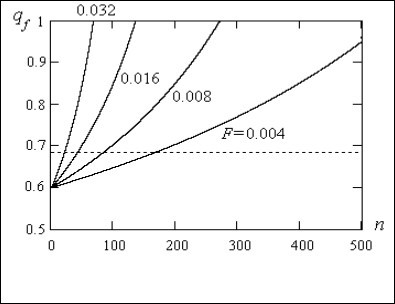From graphs it is visible that at presence of inbreeding in the population there is continuous increase of the recessive allele a frequency qf in which can arise lethal adverse (at association in homozygotes) mutations i.e. there is the negative genetic load in the population. The growth of the recessive allele frequency received in calculation is observed due to growth of the recessive homozygotes aa frequency. Thus the frequency of a heterozygotes Аа at inbreeding falls that leads to the general falling of the intact recessive allele a frequency qf on all zygotes in a population (АА,Аа,аа) due to adverse mutations only the recessive homozygotes aa.

Traditionally 8 the genetic load is estimated on the basis of the analysis of deadborns or children’s death rate with the help of two parameters A and B included in the approximate equation for the fraction of the survived zygotes:

S = exp (A+BF) ……..(30)

Unfortunately the data on experimental sizes of parameters A and B have very much variation of the values. In 1 cited the data on the population of FranceB = 1.5 - 2.5 and B/A = 15.06 - 21.41.

More detailed data are available on the population of Japan. In different prefectures the ratio B/A = 15.2-(-5). Negative value B/A means that children’s death rate in consanguineous marriages was lower than in non consanguineous marriages. Average sizes on the country A = 0.1036, B = 0.67 and B/A = 6.7

It is possible to assume that there is some threshold of a recessive homozygous genes allowable frequency compatible with a survival of individual.

Taking into account values A and B, and also inbreeding factor for Japan F = 0.004 1 under the formula (30) we shall find the fraction of the survived zygotes S = 0.9. Fraction of the perish zygotes there is 1-S = 0.1. The elementary estimation formula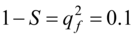gives the fraction of lethal recessive alleles at association theirs in homozygous

qf ≈ 0.316 4. Hence the fraction of not mutant genes equal to threshold frequency of the recessive alleles is qf max = 1-qf =0.684.

On Figure 2 dotted line shows the threshold frequency. For Japan this frequency is attained approximately in 167 generation.

The received result shows that accumulation of the genetic load it is process very slow. Achievement of the threshold frequency for Japan to be carried out only in 167 generations or 4175 years at time of the one generation alternation T = 25 years.

In 1 it is noticed that from the beginning of the population development with inbreeding factor F = 0.003 - 0.005 up to achievement of an equilibrium frequency which is established at action of selection should pass about 4500 years. In calculation the formula of linear increase of recessive alleles frequency was used at mutation speed μ = 10-5. Increase of the mutant alleles number was compensated by selection against recessive homozygotes with parameter of selection 0.5.

Therefore, most likely that limiting values of the genetic load in a human population of many countries especially taking into account small sizes of inbreeding factor (Canada F = 45.10-5, France F = 23.10-5, Germany F = 19.10-5, Italy F = 16.10-51 etc.) even at presence of selection till now are not achieved.

In our opinion with help of the offered method it is possible to examine not only harmful for the population genetic load related with inbreeding but also useful and indifferent genetic load (or attributes).

At early stages of mankind development the role of inbreeding was more in connection with smaller number of people and isolation of separate groups. Therefore, accumulation of the genetic load was more intensively (the inbreeding factor was much more). Primeval people after the going out from Africa and moving in northern Europe began to live in conditions of smaller light exposure including in the ultra-violet part of spectrum. In result the skin with originally dark pigmentation started to have white color. It promoted occurrence of vitamin D which deficiency results in rickets 1. Process of dark pigmentation reduction of skin can be examined as accumulation of the recessive useful genetic load. Natural selection fixed given phenotypic attribute at people in region.

To the indifferent genetic load in population it is possible to ascribe occurrence such recessive attribute as blond hair, for example, in northern part of Europe where apparently at early stages the inbreeding has been especially widespread. Color of hair does not play a role in survival of population. Fixed of this attribute was carried out by the sexual selection i.e. marriage preferences of people in region.

However it is traditionally accepted to examine accumulation of the genetic load in the population are determined by harmful mutations. Some fears of H. J. Muller are connected to this process about danger of the future biological degeneration of mankind 6.

## Migration of Inbred Populations

As it was already specified above in primitive populations, for example, leaving of Africa and moving aside northern Europe influence of inbreeding was important. Therefore, we shall consider influence of inbreeding on a migrating population.

The way of the inbreeding account for a motionless population has been analyzed in paragraph 3, equation (28). Adding in the equation (28) for allele frequency qf motionless inbred populations the item reflecting movement of a population 9 we shall find the general equation for a condition of genome in a moving inbred population: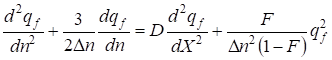…...(31)

The found equation is the nonlinear differential equation of the second order with square-law nonlinearity. qf = f (ς) We shall search the solution of the equation (31) as a traveling wave ς = kX - ωn (where In this case the equation (31) will be transformed to the kind: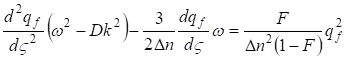…..(32)

Let’s lower the order of the equation (32) having designated.

We shall receive Abel’s differential equation: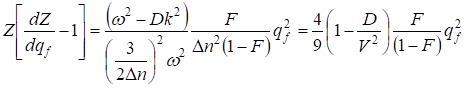…..(33)

Where ω/k = V there is speed of the population movement. Let’s note that the received differential equation does not depend on size Δn.

The analytical solution of the equation (33) does not exist. However the greatest interest has dependence of the population movement speed V on inbreeding factor F. This dependence can be found without the solving of the equation (33).

Let's consider the right part of the equation (33) which defines the inbreeding in a population. The inbreeding factor F is present both at numerator, and in the denominator. Factor F serves in numerator for establishment of all right part existence in the equation (33). At F = 0 the right part of the equation (33) disappears and the population to become panmictic i.e. in this case qf = pf0 = const. If F 0 the recessive allele frequency qf grows, Figure 2, i.e. inbreeding factor in numerator is responsible for dynamics of the function qf growth. Therefore, the inbreeding factor in numerator of the equation (33) right part should be kept at any transformations.

Taking into account the given position we shall transform the right part (33) as follows: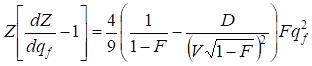…….(34)

All parameters connected to the wave phase are present only at the second addend in brackets of the right part (34). Therefore, it is possible to conclude that for the account of the inbreeding factor influence on the moving population speed it is necessary to multiply the speed of the population wave in √(1- F ).

Using result received in 9 for the genome speed V of moving population and itself population we can transform it to the kind: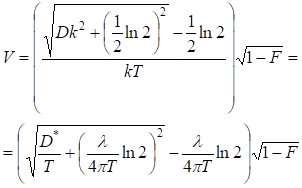……..(35)

On Figure 3 the dependence of the moving population speed on inbreeding factor is shown at the following parameters: 𝞴 = 0.4 km, D* = 10km2/year, T≈25 years.

Figure 3. Dependence of velocity of migrating population on inbreeding factor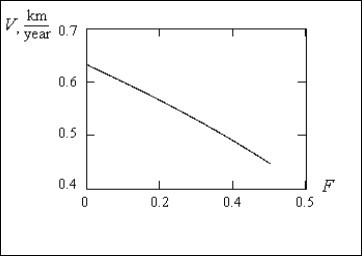The received result, Figure 3, can be interpreted as follows. At increase of inbreeding in the moving population its speed of movement decreases. Biologically it can be connected by that in the population with consanguineous mating the individuals become more weakened and during moving by less dynamical.

At F = 1 according to (35) the population stops. The reason of this stop there is fast and full degeneration of the population. If to address to the equation (28) for the motionless population (or (31) at D = 0) at F 1 the right part of the equation which determines the inbreeding quickly grows aspiring to infinity. This increase can be compensated only to increase in velocity of frequency qf growth in the left part of the equation (28). But this speed is limited by the biologo-reproductive opportunities of the population. Therefore, in a reality achievement F = 1 does not occur because the population quickly degenerates.

During migration of populations owing to small number of individuals the role of consanguineous mating was great. The found differential equation (31) of the moving inbred populations allows to analyse reduction in speed V of a population movement depending on inbreeding factor.

## Migration of Inbred Populations at Presence of Selection

The population is in process of migration usually enough long time. During migration of a population there is an alternation of generations and natural selection operates.

Using (31) and result received in 10, it is possible to write down the differential equation migrating inbred populations in which natural selection operates.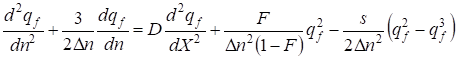……….(36)

The received nonlinear equation is difficult for the analysis. However, taking into account cubic nonlinearity of the differential equation of the second order it is possible to assume presence of solutions as solitary population waves 11. Apparently, the migrating population represents such solitary wave.

However, the equation (36) allows receive very important analytical result. For this purpose it is used wave substitution ς = 1/Δn2 (kX - ωn) where k there is wave number, ω - dimensionless cyclic frequency of the population waves. In result we shall find: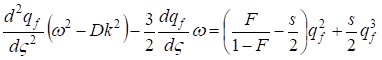……..(37)

Let’s find the particular solution of the equation (37). We shall equate the right part of this equation to zero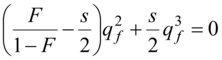. In result we have:

In result we have: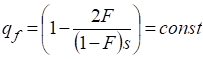…….(38)

Obviously, value of frequency qf (or pf = 1 – qf = 2F/(1-F)s ) in the formula (38) satisfies to the equations (36) and (37).

The received expression (38) can be interpreted as follows. If in inbred populations which began to migrate there is some frequency of a recessive allele in Х-chromosomes at women for preservation of this frequency should be interrelation (38) between inbreeding factor F, parameter of selection s and this frequency qf. Formula (38) is correct and for motionless populations.

We assume the frequency of the recessive allele is equal qf = 0.6. If to use inbreeding factor F = 19.10-5, for example, for Germany 1 that for a migrating population this frequency did not vary the parameter of selection must be s = 9.6.10-4.

The problem of interaction of populations is very important. Obviously, it is connected to a finding of the multiwave solutions of the equation (36) that is extremely complex mathematical problem. Population waves can cooperate with each other in the various ways.

## Population Waves can have Character of a Solitons 11.

Solitons - it is stable nonlinear solitary waves which at interaction with other local perturbations or with each other show particle like properties. For example, they restore the initial form, i.e. interactions similarly to absolutely elastic particles, for example, to absolutely elastic spheres. As well as spheres the solitons, for example, can be reflected from a barrier. The marked properties of solitons are caused they has the strict balance of the nonlinear processes resulting in increase of a steepness of a wave forward front and dispersive processes lead to destruction a solitary wave, i.e. to reduction of a front steepness. The balance of these processes leads to stability of soliton, i.e. invariability of its form.

We shall note that the third degree of nonlinearity of the differential equation (36) is sufficient for occurrence of a soliton 11. At interaction of the solitons the population waves can change directions on opposite on type impacting spheres. I.e. populations try to avoid the destruction. If populations belong to poorly cooperating individuals (elephants and birds) waves of populations at interaction pass through each other practically not influencing one on another.

As a whole, the further analysis of interaction of populations has no genetic-mathematical character and passes to area of the social phenomena.

## Conclusion

Inbreeding in a family tree and a population it is possible to take into account with the help of an inbreeding factor which is probability that two alleles at various individuals are identical by origin.

The Hardy – Weinberg’s law has a various kind for inbred a family tree and populations. In a family tree the inbreeding factor is included into the discrete equation, and populations in the differential equation. The numerical solution of this differential equation is found.

Found the distribution of genotypes in Х-chromosomes shows that as well as in autosomes at inbreeding the fraction of homozygotes increased, and the fraction of heterozygotes is reduced. At the greater predisposition to mutations of the recessive homozygotes which frequency increases at inbreeding the accumulation of a genetic load is possible.

It is shown that speed of a migrating population at increase of inbreeding factor is reduced.

Interrelation of the recessive allele frequency at woman for a migrating population with inbreeding factor and standard parameter of selection was found.